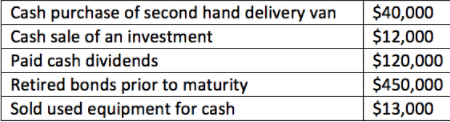# Problem: Consider the following transactions: What was the net cash flow from investing activities? A. $27,000 inflow B.$330,000 outflow C. $15,000 outflow D.$585,000 outflow

###### Problem Details

Consider the following transactions:

What was the net cash flow from investing activities?

A. $27,000 inflow B.$330,000 outflow

C. $15,000 outflow D.$585,000 outflowFrequently Asked Questions

What scientific concept do you need to know in order to solve this problem?

Our tutors have indicated that to solve this problem you will need to apply the Investing Activities concept. You can view video lessons to learn Investing Activities. Or if you need more Investing Activities practice, you can also practice Investing Activities practice problems.

What is the difficulty of this problem?

Our tutors rated the difficulty ofConsider the following transactions: What was the net cash ...as medium difficulty.